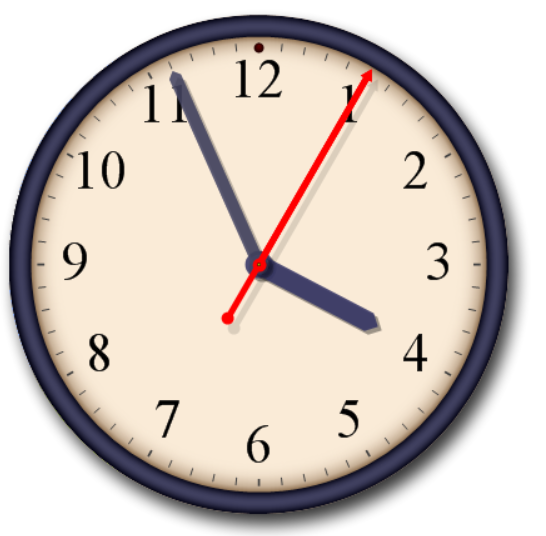# Definition of Minute HandThe minute hand is the long black hand on a clock that shows how many minutes past the hour it is.

The minute hand goes around the clock once every hour.

For example, the minute hand in the clock is pointing to the first mark after the $11$. This tells us that it is $56$ minutes past the hour, or $4$ minutes to the next hour.

The shorter, fatter hand on the clock is the hour hand. It is almost pointing to $4$ o'clock, so we know the time is $4$ minutes to $4$ o'clock. The hour hand takes 12 hours to go around the clock once.

The thin red hand is the second hand. Not all clocks have this. The second hand once around the clock every minute.

### Description

The aim of this dictionary is to provide definitions to common mathematical terms. Students learn a new math skill every week at school, sometimes just before they start a new skill, if they want to look at what a specific term means, this is where this dictionary will become handy and a go-to guide for a student.

### Audience

Year 1 to Year 12 students

### Learning Objectives

Learn common math terms starting with letter M

Author: Subject Coach
You must be logged in as Student to ask a Question.